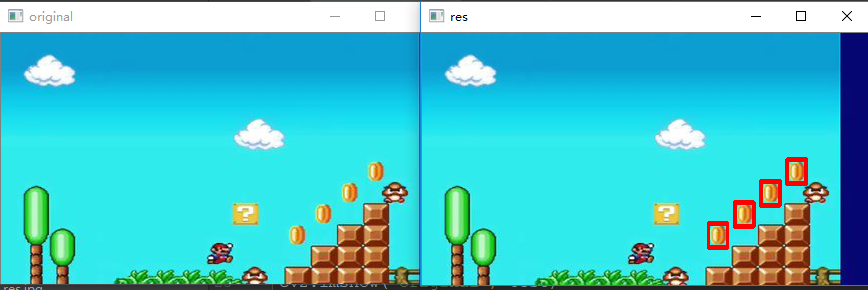# 图像处理模板匹配

## 图像处理（十）：模板匹配

2018-07-05 15:24:20 sinat_31425585 阅读数 5843
• ###### OpenCV3.2 Java图像处理视频学习教程

OpenCV3.2 Java图像处理视频...主要内容包括opencv像素操作、滤波、边缘提取、直线与圆检测、形态学操作与分水岭、图像金子塔融合重建、多尺度模板匹配、opencv人脸检测、OpenCV跟Tomcat使用实现服务器端图像处理服务。

39课时 884分钟 4656人学习 贾志刚
免费试看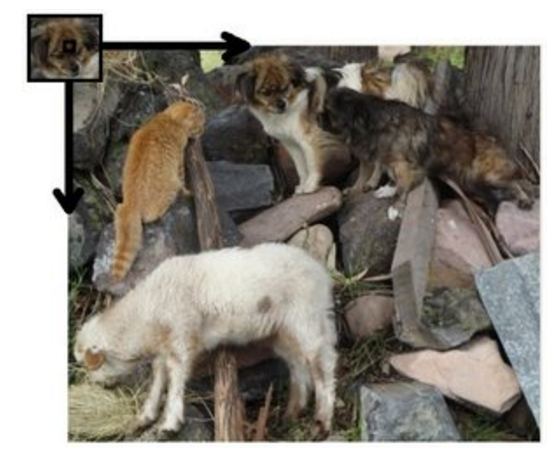左上角小狗图像为定义的模板，下面的图片为待匹配图像，匹配的过程就是按照从左到右，从上到下的顺序，依次遍历全图，通过定义的相似度评判准则，计算模板与当前位置图像块的相似度，进而得到最相似位置，实现目标定位。

常见的相似度准则有：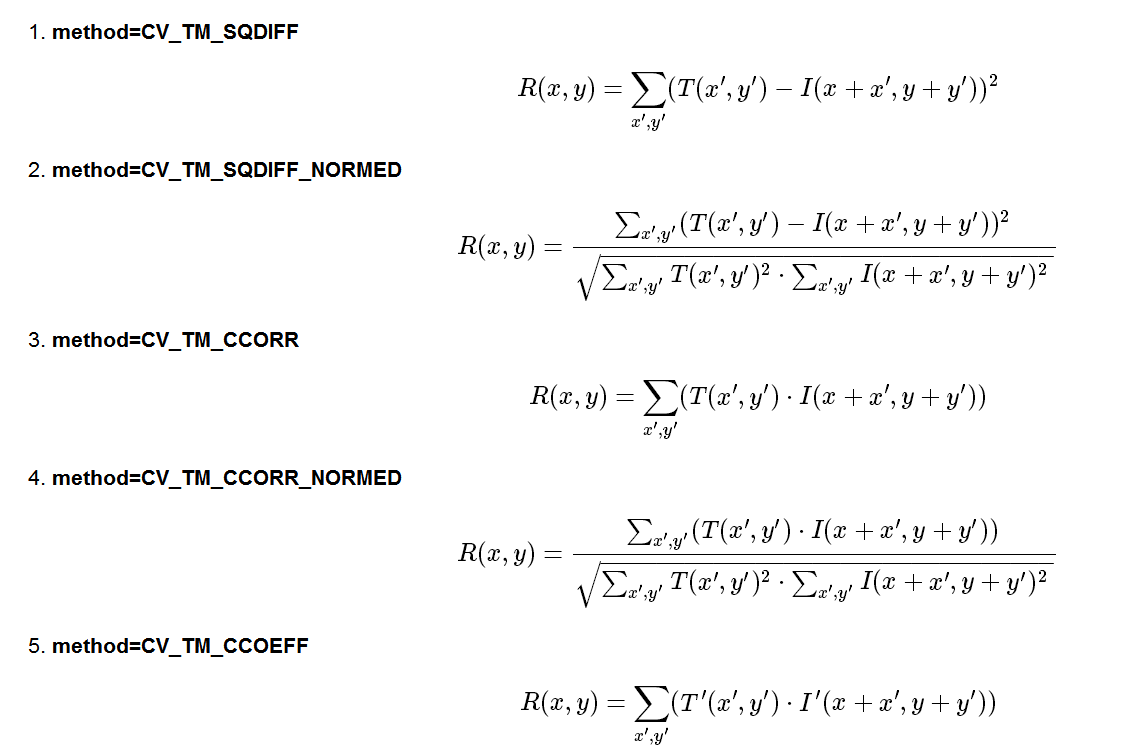即：平方差、归一化平方差、相关系数、归一化相关系数、互相关五种。

下面就是代码，这里只实现了两种：平方误差及绝对误差

``````#include <opencv2/opencv.hpp>
#include <math.h>
#include <iostream>

using namespace cv;
using namespace std;

#define SQDIFF 0

float templateMatch(const Mat & src, const Mat & temp, int & i_match, int & j_match, int Match_methold)
{
int src_cols = src.cols;
int src_rows = src.rows;
int temp_cols = temp.cols;
int temp_rows = temp.rows;

int i_end = src_rows - temp.rows + 1;
int j_end = src.cols - temp.cols + 1;

float match_degree = FLT_MAX;

for (int i = 0; i < i_end; i++)
{
for (int j = 0; j < j_end; j++)
{
float match_ij = 0.0;

for (int m = 0; m < temp_rows; m++)
{
for (int n = 0; n < temp_cols; n++)
{
uchar val_s = src.at<uchar>(i + m, j + n);
uchar val_t = temp.at<uchar>(m, n);
if (Match_methold == SQDIFF)
{
match_ij += float((val_t - val_s) * (val_t - val_s));
}
{
match_ij += float(abs(val_t - val_s));
}
}
}

//cout << match_ij << endl;
if (match_ij < match_degree)
{
match_degree = match_ij;
i_match = i;
j_match = j;
}
}
}

return match_degree;

}

int main()
{

float match_degree = 0;
int i_match = -1, j_match = -1;
match_degree = templateMatch(src, temp, i_match, j_match, SQDIFF);

cout << i_match << j_match << match_degree << endl;

Mat src_color;
cvtColor(src, src_color, COLOR_GRAY2BGR);
rectangle(src_color, Rect(j_match, i_match, temp.cols, temp.rows), Scalar(255, 0, 0));
imshow("result", src_color);
waitKey(0);

return 0;
}``````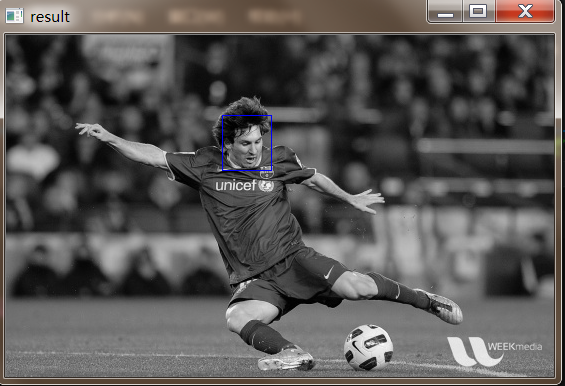1）增加步长

2）随机抽样匹配

增加步长就不多讨论了，随机抽样，利用的是目标所在矩形区域位置中，目标应该位于矩形区域中央，边界部分大部分为背景，因此考虑对模版进行随机采样，采样方法为：越靠近中心位置，生成越多的采样点，反之，越远离中心位置，生成较少的采样点，这里采用的随机生成函数为Gaussian函数。

``````int main()
{

float match_degree = 0;
int i_match = -1, j_match = -1;

vector <Point2i> real_sample_points;
computeSamplePoints(real_sample_points, Rect(0, 0, temp.cols - 1, temp.rows - 1));

match_degree = templateMatch(src, temp, i_match, j_match, SQDIFF, real_sample_points);

cout << i_match << j_match << match_degree << endl;

Mat src_color;
cvtColor(src, src_color, COLOR_GRAY2BGR);
for (int i = 0; i < 1000; i++){
circle(src_color, Point(j_match, i_match) + real_sample_points[i], 1, Scalar(0, 255, 0));
}
rectangle(src_color, Rect(j_match, i_match, temp.cols, temp.rows), Scalar(255, 0, 0));
imshow("result", src_color);
waitKey(0);

return 0;

}``````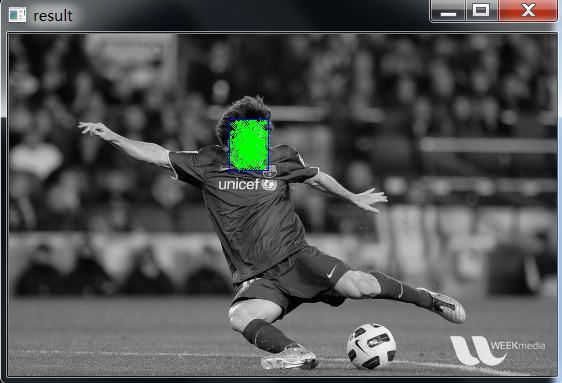opencv 官网

## 图像处理笔记（十八）：模板匹配

2019-07-12 18:11:00 weixin_30655569 阅读数 72
• ###### OpenCV3.2 Java图像处理视频学习教程

OpenCV3.2 Java图像处理视频...主要内容包括opencv像素操作、滤波、边缘提取、直线与圆检测、形态学操作与分水岭、图像金子塔融合重建、多尺度模板匹配、opencv人脸检测、OpenCV跟Tomcat使用实现服务器端图像处理服务。

39课时 884分钟 4656人学习 贾志刚
免费试看

``````dev_close_window ()
dev_open_window_fit_image(Image, 0, 0, 512, 512, WindowHandle)
dev_display(Image)

* 从原图中裁切一块作为模板
crop_part(Image, ImagePart, 445, 250, 240, 220)
dev_set_draw('margin')
mean_image(ImagePart, ImageMean, 3, 3)
rgb1_to_gray(ImageMean, GrayImage)
* 将原图缩小到一半
zoom_image_size(GrayImage, GrayImage, 120, 110, 'constant')
* 使用切出来创建一个ncc模板（模板生成方式1）
create_ncc_model(GrayImage, 'auto', -0.39, 0.79, 'auto', 'use_polarity', ModelID)
rotate_image(Image, ImageRotate, 45, 'constant')
rgb1_to_gray(Image, GrayImage1)
* 匹配
find_ncc_model(GrayImage1, ModelID, -0.39, 0.79, 0.8, 1, 0.1, 'true', 0, Row, Column, Angle, Score)
if (|Row| > 0)
gen_rectangle1(Rectangle, Row - 100, Column - 100, Row + 100, Column + 100)
endif

* 模板生成方式2，这个模板是可以旋转匹配的，也就是说，如果图像旋转了，上面那个模板就匹配不到
create_ncc_model(GrayImage, 'auto', 0, rad(360), 'auto', 'use_polarity', ModelID1)
rgb1_to_gray(ImageRotate, GrayImage2)
find_ncc_model(GrayImage2, ModelID1, 0, rad(360), 0.8, 1, 0.1, 'true', 0, Row1, Column1, Angle1, Score1)
if (|Row1| > 0)
gen_rectangle2(Rectangle1, Row1, Column1, Angle1, 100, 100)
endif

* 模板生成方式3，这个模板是适合有缩放或有旋转的匹配的，如果模板进行了缩放或者图像进行了缩放，上面那两个模板都会匹配不了
* 旋转角度范围和图片缩放比例可配，如果支持的范围太大的话，会影响匹配速度。
create_scaled_shape_model(GrayImage, 'auto', 0, rad(140), 'auto', 0.5, 10, 'auto', 'auto', 'use_polarity', 'auto', 'auto', ModelID2)
find_scaled_shape_model(GrayImage2, ModelID2, 0, rad(140), 0.5, 10, 0.5, 1, 0.5, 'least_squares', 0, 0.1, Row2, Column2, Angle2, Scale, Score2)
if (|Row2| > 0)
gen_rectangle2(Rectangle2, Row2, Column2, Angle2, 100, 100)
endif``````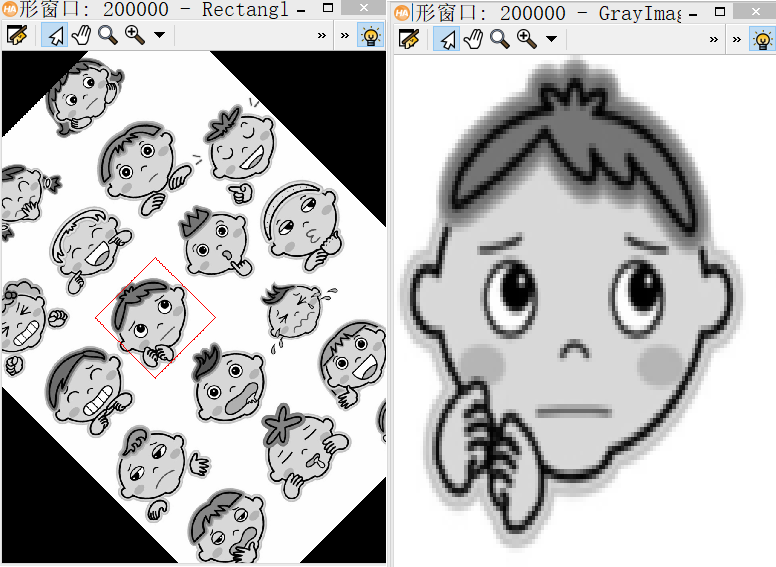create_scaled_shape_model(Template, 输出值，模板
NumLevels, 图像金字塔层数，用来降低匹配时长，每增加一层图像的宽高就会减半，图像分辨率会降低
AngleStart, 旋转开始的弧度
AngleExtend, 旋转的角度范围
AngleStep, 旋转步长，如果不知道怎么设置，可以设置为auto，步长设置的越小，越占用内存，越慢
ScaleMin, 最小缩放倍数
ScaleMax, 最大缩放倍数
ScaleStep, 缩放步长，可以设置为auto
Optimization, 优化方式
Metric, 是否使用极性，如果使用极性，那么如果模板背景灰度弱，前景灰度强，那么匹配的图片也必须满足这样的条件；如果不要求极性，当匹配的图片背景灰度强，前景灰度弱的时候也会匹配到，忽略极性会导致匹配耗时增加
Contrast, 和模板图片对比度相关的一个阈值，可以直接使用auto
MinContrast, 用来匹配的图片的最小对比度
ModelID) 输出值，模板ID

find_scaled_shape_model(Image, 用来查找的图片
ModelID, 模板ID
AngleStart,
AngleExtent,
ScaleMin,
ScaleMax,
MinScore, 匹配的最低分数，大于这个分数的才是被接受的匹配
NumMatches, 需要找的匹配数量
MaxOverlap, 最大重叠
SubPixel, 是否亚像素精度
NumLevels, 图像金字塔层数
Greediness, 搜索贪婪度，0-1，越大搜索越快，可以理解为搜得越不仔细
Row, 搜索到的行坐标
Column, 搜索到的列坐标
Angle, 相对于x轴正半轴的旋转角度
Scale, 图片相对于模板的缩放倍数bc
Score) 得分

## 图像处理 模板匹配

2019-06-01 20:57:00 Yoci98 阅读数 170
• ###### OpenCV3.2 Java图像处理视频学习教程

OpenCV3.2 Java图像处理视频...主要内容包括opencv像素操作、滤波、边缘提取、直线与圆检测、形态学操作与分水岭、图像金子塔融合重建、多尺度模板匹配、opencv人脸检测、OpenCV跟Tomcat使用实现服务器端图像处理服务。

39课时 884分钟 4656人学习 贾志刚
免费试看

VS2017 + OpenCV4.0.1编译

``` 1 #include <iostream>
2 #include <opencv2/opencv.hpp>
3 #include <opencv2/core.hpp>
4 #include <opencv2/highgui.hpp>
5 #include <opencv2/imgproc.hpp>
6 using namespace std;
7 using namespace cv;
8
9 //定义全局变量
10 Mat src, temp, res;
11
12 //显示窗口名
13 string img_window = "source image";
14 string res_window = "result image";
15
16 int match_method;
17 int max_tracker = 5;
18
19 //滑动条回调函数
20 void match(int pos, void*)
21 {
22     Mat subImg;
23     src.copyTo(subImg);//拷贝副本
24     int res_row = src.rows - temp.rows + 1;
25     int res_col = src.cols - temp.cols + 1;
26     res.create(res_row, res_col, CV_32FC1);//创建结果矩阵
27
28     //模板匹配
29     matchTemplate(subImg, temp, res, match_method);
30     normalize(res, res, 0, 1, NORM_MINMAX, -1, Mat());
31
32     //定位
33     double minVal, maxVal;
34     Point minLoc, maxLoc, matchLoc;
35     minMaxLoc(res, &minVal, &maxVal, &minLoc, &maxLoc, Mat());
36
37     //分类讨论
38     if (match_method == TM_SQDIFF || match_method == TM_SQDIFF_NORMED)
39         matchLoc = minLoc;
40     else
41         matchLoc = maxLoc;
42
43     //矩形框显示匹配结果
44     rectangle(subImg, matchLoc, Point(matchLoc.x + temp.cols, matchLoc.y + temp.rows), Scalar(0, 255, 0), 2);
45     rectangle(res, matchLoc, Point(matchLoc.x + temp.cols, matchLoc.y + temp.rows), Scalar(0, 255, 0), 2);
46
47     imshow(img_window, subImg);
48     imshow(res_window, res);
49 }
50
51 int main()
52 {
53     //读入原图和模板图
56     imshow("模板", temp);
57
58     //创建显示窗口
59     namedWindow(img_window);
60     namedWindow(res_window);
61
62     //创建滑动条
63     match(2, 0);
64     createTrackbar("匹配方法", img_window, &match_method, max_tracker, match);
65
66     waitKey(0);
67     return 0;
68 }```

## 图像处理中的模板匹配c++实现

2017-08-16 22:05:16 wu_lian_nan 阅读数 5087
• ###### OpenCV3.2 Java图像处理视频学习教程

OpenCV3.2 Java图像处理视频...主要内容包括opencv像素操作、滤波、边缘提取、直线与圆检测、形态学操作与分水岭、图像金子塔融合重建、多尺度模板匹配、opencv人脸检测、OpenCV跟Tomcat使用实现服务器端图像处理服务。

39课时 884分钟 4656人学习 贾志刚
免费试看

• 基于相关的模板匹配技术可直接用于在一幅图像中，寻找某种子图像模式。对于大小为MxN的图像f(x,y)和大小为JxK的子图像模式w(x,y),f与w的相关可表示为：• 其种，x=0,1,2,…N-K,y=0,1,2,…M-J。此处
的目的是寻找匹配而不是对f(x,y)进行滤波操作，因此w的原点被设置在子图像的左上角，
并且式(11-3) 给出的形式也完全适用于J和K 为偶数的情况。

• 计算相关c(x,y)的过程就是在图像f(x,y)中逐点地移动子图像w(x,小),使w 的原点和点
(x,y)重合，然后计算w与f中被w覆盖的图像区域对应像素的乘积之和，以此计算结果作
为相关图像c(x,)在(x,y)点的响应。

• 相关可用于在图像f(x,y)中找到与子图像w(x,y)匹配的所有位置。实际上，当w 按照上
段中描述的过程移过整幅图像f之后，最大的响应点(xo,yo)即为最佳匹配的左上角点。我们
也可以设定一个阈值T,认为响应值大于该阈值的点均是可能的匹配位置。

• 相关的计算是通过将图像元素和子模式图像元素联系起来获得的，将相关元素相乘后累
加。我们完全可以将子图像w视为一个按行或按列存储的向量，将计算过程中被w覆盖的图像区域视为另一个按照同样的方式存储的向量.这样一来,相关计算就- 成了向量之间的点积运算。• 其中，Θ 为向量之间的夹角。显然，当具有完全相同的方向(平行) 时，,从而式(11-4) 取得其最大值，这就意味着当图像的局部区域类似于子图像模式时，相关运算产生最大的响应。然而，式(11-4) 最终的取值还与向量自身的模有关，这将导致按照式(11-4) 计算的相关响应存在着对f和w 的灰度幅值比较敏感的缺陷。这样一来，在f的高灰度区域，可能尽管其内容与子图像w的内容并不相近，但由于自身较大而同样产生一个很高的响应。可通过对向量以其模值来归一化解决这一问题，即通过来计算。• 看了脑疼的理论，下面看看让人愉快的代码吧。

TemplateMatch.h

``````#pragma once
#include<opencv2\opencv.hpp>
using namespace cv;
typedef unsigned char BYTE;
void TemplateMatch(Mat * pTo, Mat * pTemplate,Mat * src);
``````

TemplateMatch.cpp

`````` #include"TemplateMatch.h"

void TemplateMatch(Mat * pTo, Mat * pTemplate, Mat * src)
{

//循环变量
int i, j, m, n;

double dSumT; //模板元素的平方和
double dSumS; //图像子区域元素的平方和
double dSumST; //图像子区域和模板的点积

//响应值
double R;

//记录当前的最大响应
double MaxR;

//最大响应出现位置
int nMaxX;
int nMaxY;

int nHeight = src->rows;
int nWidth = src->cols;
//模板的高、宽
int nTplHeight = pTemplate->rows;
int nTplWidth = pTemplate->cols;

//计算 dSumT
dSumT = 0;
for (m = 0; m < nTplHeight; m++)
{
for (n = 0; n < nTplWidth; n++)
{
// 模板图像第m行，第n个象素的灰度值
int nGray =*pTemplate->ptr(m, n);

dSumT += (double)nGray*nGray;
}
}

//找到图像中最大响应的出现位置
MaxR = 0;
for (i = 0; i < nHeight - nTplHeight + 1; i++)
{
for (j = 0; j < nWidth - nTplWidth + 1; j++)
{
dSumST = 0;
dSumS = 0;

for (m = 0; m < nTplHeight; m++)
{
for (n = 0; n < nTplWidth; n++)
{
// 原图像第i+m行，第j+n列象素的灰度值
int nGraySrc = *src->ptr(i + m, j + n);

// 模板图像第m行，第n个象素的灰度值
int nGrayTpl = *pTemplate->ptr(m, n);

dSumS += (double)nGraySrc*nGraySrc;
dSumST += (double)nGraySrc*nGrayTpl;
}
}

R = dSumST / (sqrt(dSumS)*sqrt(dSumT));//计算相关响应

//与最大相似性比较
if (R > MaxR)
{
MaxR = R;
nMaxX = j;
nMaxY = i;
}
}
}

//将找到的最佳匹配区域复制到目标图像
for (m = 0; m < nTplHeight; m++)
{
for (n = 0; n < nTplWidth; n++)
{
int nGray = *src->ptr(nMaxY + m, nMaxX + n);
//pTo->setTo(nMaxX + n, nMaxY + m, RGB(nGray, nGray, nGray));
pTo->at<BYTE>(nMaxY + m, nMaxX + n) = nGray;
}
}

}   ``````

``````#include"TemplateMatch.h"
#include<iostream>

using namespace std;
int main()
{
Mat pt=src;
pt.data = new BYTE[src.cols*src.rows];
memset(pt.data, 255, src.cols*src.rows);
TemplateMatch(&pt, &Template, &src);
imshow("S", src);
imshow("T", Template);
imshow("P", pt);

imwrite("S.jpg", src);
imwrite("T.jpg", Template);
imwrite("P.jpg", pt);
waitKey(0);
return 0;
}````````````    下面给出这个工程的百度云连接：链接：http://pan.baidu.com/s/1jIuuT3w 密码：veto
``````

## (三)OpenCV中的图像处理之图像变换及模板匹配

2018-05-30 15:31:55 u014403318 阅读数 3120
• ###### OpenCV3.2 Java图像处理视频学习教程

OpenCV3.2 Java图像处理视频...主要内容包括opencv像素操作、滤波、边缘提取、直线与圆检测、形态学操作与分水岭、图像金子塔融合重建、多尺度模板匹配、opencv人脸检测、OpenCV跟Tomcat使用实现服务器端图像处理服务。

39课时 884分钟 4656人学习 贾志刚
免费试看

### 3.10 OpenCV中的图像变换

1.目标

• 使用OpenCV查找图像的傅里叶变换
• 利用Numpy中可用的FFT函数
• 傅里叶变换的一些应用
• 我们将学习以下函数：cv2.dft()、cv2.idft()等

2.原理

3.Numpy中的傅里叶变换

```import cv2
import numpy as np
from matplotlib import pyplot as plt

f = np.fft.fft2(img)  # 快速傅里叶变换算法得到频率分布
fshift = np.fft.fftshift(f)  # 默认结果中心点位置是在左上角，转移到中间位置
magnitude_spectrum = 20 * np.log(np.abs(fshift))  # 结果是复数，求绝对值才是振幅

# 结果展示
plt.subplot(121), plt.imshow(img, 'gray')
plt.title('Input Image'), plt.xticks([]), plt.yticks([])
plt.subplot(122), plt.imshow(magnitude_spectrum, 'gray')
plt.title('Magnitude Spectrum'), plt.xticks([]), plt.yticks([])
plt.show()```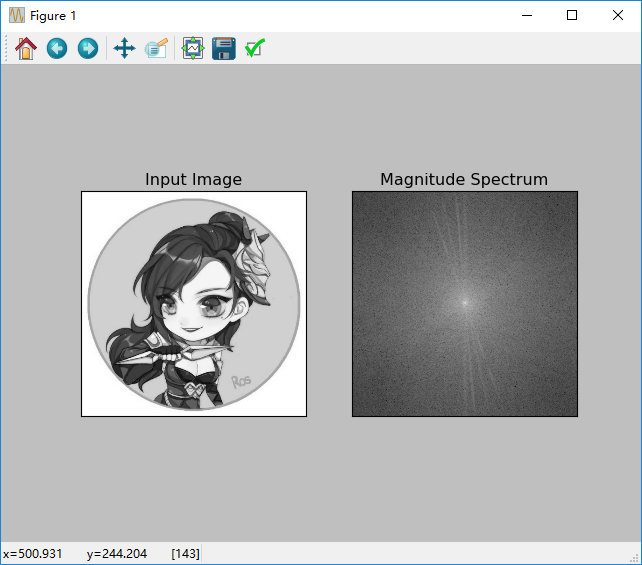```import cv2
import numpy as np
from matplotlib import pyplot as plt

f = np.fft.fft2(img)  # 快速傅里叶变换算法得到频率分布
fshift = np.fft.fftshift(f)  # 默认结果中心点位置是在左上角，转移到中间位置
magnitude_spectrum = 20 * np.log(np.abs(fshift))  # 结果是复数，求绝对值才是振幅

# 找到频域变换后，可以在频域中做一些操作，如高通滤波和重构图像，即找到逆DFT
rows, cols = img.shape
crow, ccol = rows / 2, cols / 2
fshift[crow - 30:crow + 30, ccol - 30:ccol + 30] = 0
f_ishift = np.fft.ifftshift(fshift)
img_back = np.fft.ifft2(f_ishift)
img_back = np.abs(img_back)

plt.subplot(131), plt.imshow(img, 'gray')
plt.title("Input Image"), plt.xticks([]), plt.yticks([])
plt.subplot(132), plt.imshow(img_back, 'gray')
plt.title('Image After HPF'), plt.xticks([]), plt.yticks([])
plt.subplot(133), plt.imshow(img_back)
plt.title('Result in JET'), plt.xticks([]), plt.yticks([])

plt.show()```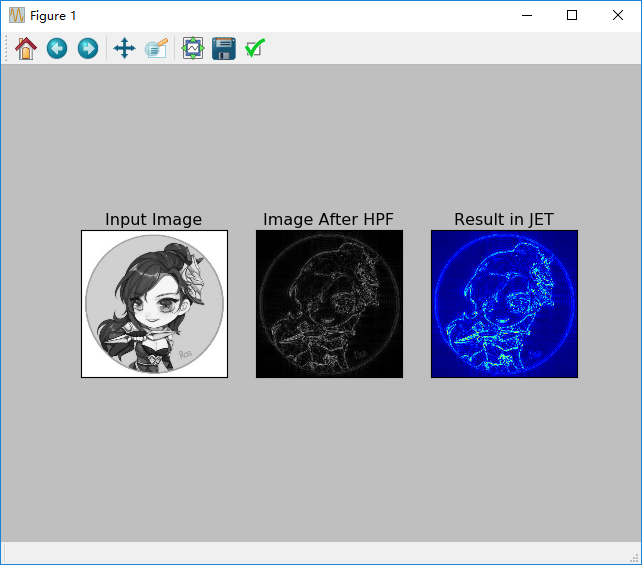4.OpenCV中的傅里叶变换

OpenCV为此提供了cv2.dft()cv2.idft().它返回与以前相同的结果，但具有两个通道。第一个通道将具有结果的实际部分，第二个通道将具有结果的虚部。输入图像应首先转换为np.float32.我们将看到如何去做。

```# -*- coding: utf-8 -*-
'''
OpenCV中的傅里叶变换：
1.cv2.dft()和cv2.idft(),输入图像应先转换成np.float32
attention:也可以使用cv2.cartToPolar(),可以一次性返回幅值和相位
cv2.dft()和cv2.idft()比Numpy对应的函数更快，但是Numpy函数更友好。
'''

import cv2
import numpy as np
from matplotlib import pyplot as plt

dft = cv2.dft(np.float32(img), flags=cv2.DFT_COMPLEX_OUTPUT)
dft_shift = np.fft.fftshift(dft)

magnitude_spectrum = 20 * np.log(cv2.magnitude(dft_shift[:, :, 0], dft_shift[:, :, 1]))
'''
plt.subplot(121), plt.imshow(img, 'gray')
plt.title('Input Image'), plt.xticks([]), plt.yticks([])
plt.subplot(122), plt.imshow(magnitude_spectrum, 'gray')
plt.title('Magnitude Spectrum'), plt.xticks([]), plt.yticks([])
plt.show()
'''

rows, cols = img.shape
crow, ccol = int(rows / 2), int(cols / 2)  # 这里还是必须转换为整数哦

# 首先创建一个掩膜，中心点为1，其余值为0
mask = np.zeros((rows, cols, 2), np.uint8)
mask[crow - 30:crow + 30, ccol - 30: ccol + 30] = 1

# 应用掩膜和逆DFT
f_ishift = np.fft.ifftshift(fshift)
img_back = cv2.idft(f_ishift)

img_back = cv2.magnitude(img_back[:, :, 0], img_back[:, :, 1])
plt.subplot(121), plt.imshow(img, 'gray')
plt.title('Input Image'), plt.xticks([]), plt.yticks([])
plt.subplot(122), plt.imshow(img_back, 'gray')
plt.title('Magnitude Spectrum'), plt.xticks([]), plt.yticks([])

plt.show()
```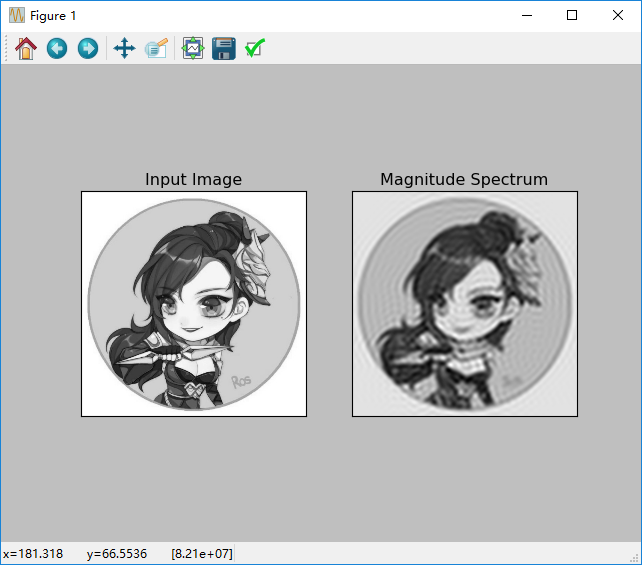5.DFT的性能优化

`In : img = cv2.imread('messi5.jpg',0)`
`In : rows,cols = img.shape`
`In : print rows,cols`
`342 548`
` `
`In : nrows = cv2.getOptimalDFTSize(rows)`
`In : ncols = cv2.getOptimalDFTSize(cols)`
`In : print nrows, ncols`
`360 576`

`nimg = np.zeros((nrows,ncols))`
`nimg[:rows,:cols] = img`

`right = ncols - cols`
`bottom = nrows - rows`
`bordertype = cv2.BORDER_CONSTANT #just to avoid line breakup in PDF file`
`nimg = cv2.copyMakeBorder(img,0,bottom,0,right,bordertype, value = 0)`

`In : %timeit fft1 = np.fft.fft2(img)`
`10 loops, best of 3: 40.9 ms per loop`
`In : %timeit fft2 = np.fft.fft2(img,[nrows,ncols])`
`100 loops, best of 3: 10.4 ms per loop`

`In : %timeit dft1= cv2.dft(np.float32(img),flags=cv2.DFT_COMPLEX_OUTPUT)`
`100 loops, best of 3: 13.5 ms per loop`
`In : %timeit dft2= cv2.dft(np.float32(nimg),flags=cv2.DFT_COMPLEX_OUTPUT)`
`100 loops, best of 3: 3.11 ms per loop`

6.为什么拉普拉斯算子是高通滤波器

```# -*- coding: utf-8 -*-
'''

'''
import cv2
import numpy as np
from matplotlib import pyplot as plt

#简单的均值滤波器没有参数
mean_filter=np.ones((3,3))

#创建一个高斯滤波器
x=cv2.getGaussianKernel(5,10)
gaussian=x*x.T

#不同的边缘检测滤波器
#x方向上的scharr
scharr=np.array([[-3,0,3],
[-10,0,10],
[-3,0,3]])

#x方向上的sobel
sobel_x=np.array([[-1,0,1],
[-2,0,2],
[-1,0,1]])

#y方向上的sobel
sobel_y=np.array([[-1,-2,-1],
[0,0,0],
[1,2,1]])

#拉普拉斯算子
laplacian=np.array([[0,1,0],
[1,-4,1],
[0,1,0]])

filters=[mean_filter,gaussian,scharr,sobel_x,sobel_y,laplacian]
filter_name=['mean_filter','gaussian','scharr','sobel_x','sobel_y','laplacian']
fft_filter=[np.fft.fft2(x) for x in filters]
fft_shift=[np.fft.fftshift(y) for y in fft_filter]
#地图谱
map_spectrum=[np.log(np.abs(z)+1) for z in fft_shift]

for i in range(6):
plt.subplot(2,3,i+1),plt.imshow(map_spectrum[i],'gray')
plt.title(filter_name[i]),plt.xticks([]),plt.yticks([])

plt.show()
```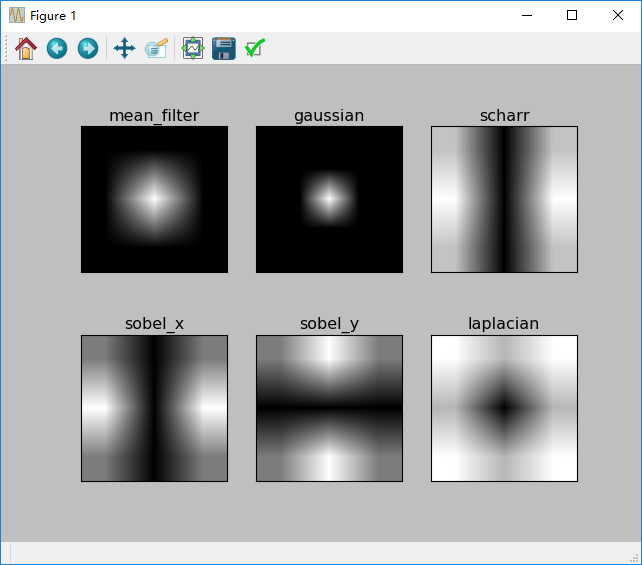### 3.11 模板匹配

1.目标

• 使用模板匹配查找图像中的对象
• 学会这些函数：cv2.matchTemplate()、cv2.minMaxLoc()

2.原理

3.OpenCV中的模板匹配```# -*- coding: utf-8 -*-
'''
OpenCV中的模板匹配
1.cv2.matchTemplate()、cv2.minMaxLoc()
2.OpenCV中实现了集中比较方法，返回一个灰度图像，其中每个像素表示该像素的领域和模板匹配的程度
3.输入图像大小若是(w*h),则输出图像大小为(W-w+1,H-h+1).一旦得到结果，可以使用cv2.minMaxLoc()函数来查找最大值和最小值

'''
import cv2
import numpy as np
from matplotlib import pyplot as plt

img2 = img.copy()

w, h = template.shape[::-1]
# 6中类型的比较
methods = ['cv2.TM_CCOEFF', 'cv2.TM_CCOEFF_NORMED', 'cv2.TM_CCORR',
'cv2.TM_CCORR_NORMED', 'cv2.TM_SQDIFF', 'cv2.TM_SQDIFF_NORMED']

for meth in methods:
img = img2.copy()
method = eval(meth)
# 应用模板匹配
res = cv2.matchTemplate(img, template, method)
min_val, max_val, min_loc, max_loc = cv2.minMaxLoc(res)

# 如果方法是cv2.TM_SQDIFF或cv2.TM_SQDIFF_NORMED
if method in [cv2.TM_SQDIFF, cv2.TM_SQDIFF_NORMED]:
top_left = min_loc
else:
top_left = max_loc

bottom_right = (top_left + w, top_left + h)
cv2.rectangle(img, top_left, bottom_right, 255, 2)

plt.subplot(121), plt.imshow(res, 'gray')
plt.title('Matching Result'), plt.xticks([]), plt.yticks([])
plt.subplot(122), plt.imshow(img, 'gray')
plt.title('Detected Point'), plt.xticks([]), plt.yticks([])
plt.suptitle(meth)

plt.show()
```

cv2.TM_CCOEFF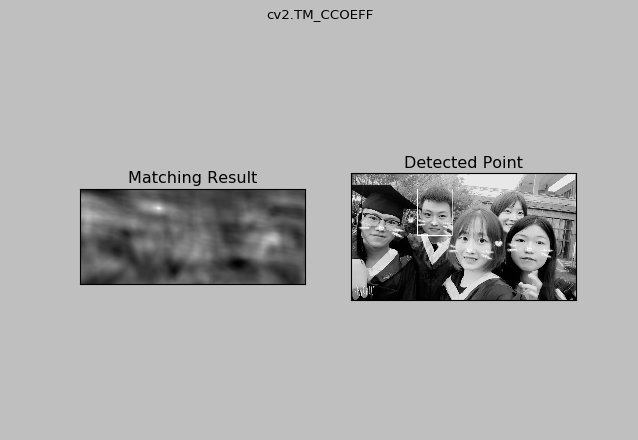cv2.TM_CCOEFF_NORMED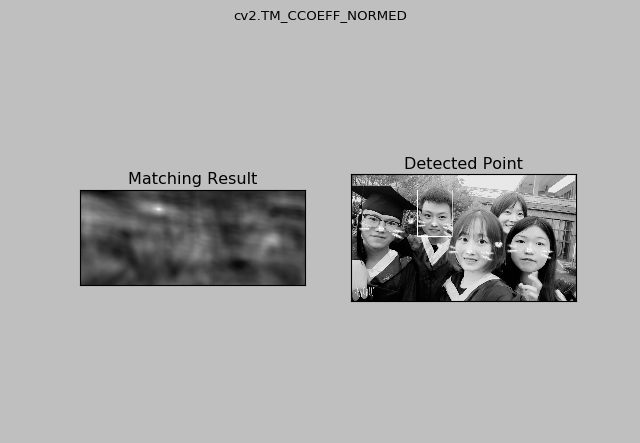cv2.TM_CCORR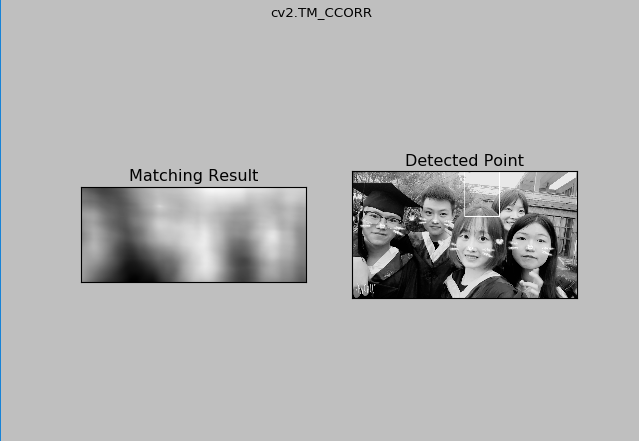cv2.TM_CCORR_NORMED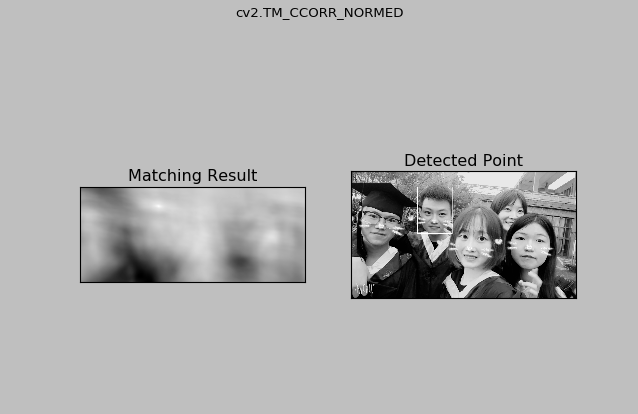cv2.TM_SQDIFF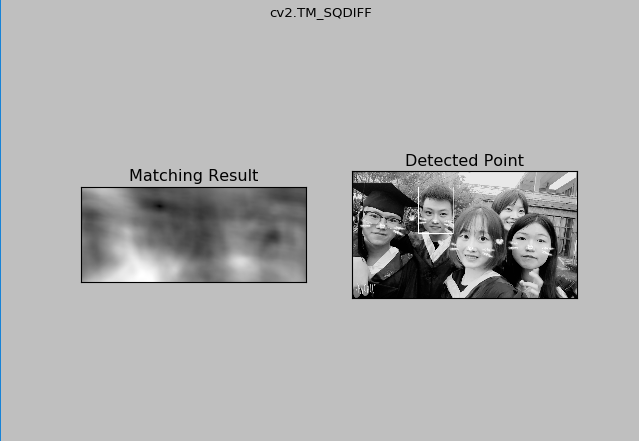cv2.TM_SQDIFF_NORMED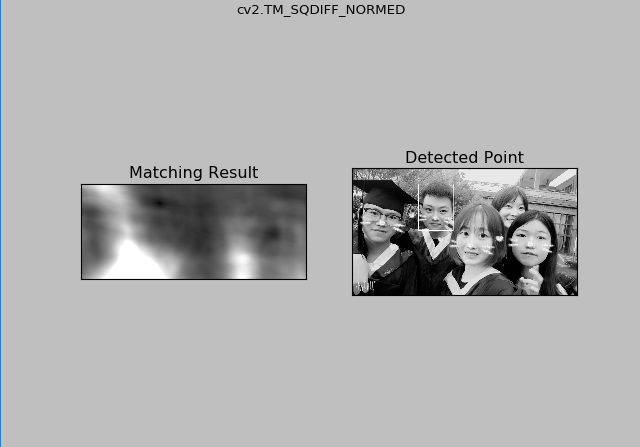4.多目标的模板匹配

```# -*- coding: utf-8 -*-
'''

'''

import cv2
import numpy as np
from matplotlib import pyplot as plt

img_gray = cv2.cvtColor(img_rgb, cv2.COLOR_BGR2GRAY)
w, h = template.shape[::-1]

res = cv2.matchTemplate(img_gray, template, cv2.TM_CCOEFF_NORMED)
threshold = 0.8
loc = np.where(res >= threshold)
for pt in zip(*loc[::-1]):
cv2.rectangle(img_rgb, pt, (pt + w, pt + h), (0, 0, 255), 2)

cv2.imwrite('res.jpg', img_rgb)
cv2.imshow('original', test)
cv2.imshow('res', img_rgb)
cv2.waitKey(0) & 0xFF
cv2.destroyAllWindows()
```